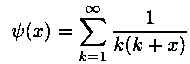# Numerical Summation of a Series

Time Limit: Java: 10000 ms / Others: 10000 ms

Memory Limit: Java: 32768 KB / Others: 32768 KB

## Description

Produce a table of the values of the seriesEquation 1

for the 2001 values of x, x= 0.000, 0.001, 0.002, ..., 2.000.All entries of thetable must have an absolute error less than 0.5e-12 (12 digits ofprecision). This problem is based on a problem from Hamming (1962), whenmainframes were very slow by today's microcomputer standards.

## Input

This problem has no input.

## Output

The output is to beformatted as two columns with the values of x andy(x)printed as in the C printf or the Pascal writeln.printf("%5.3f %16.12f\n", x, psix ) writeln(x:5:3, psix:16:12) As an example, here are 4 acceptable lines out of 2001.0.000 1.644934066848...0.500 1.227411277760...1.000 1.000000000000...2.000 0.750000000000 The values of x should start at 0.000 and increase by 0.001 until the linewith x=2.000 is output.

## Sample Input

None

## Sample Output

None

## Hint

The problem with summing the sequence in equation 1 is that too many terms may be required to complete the summation in the given time. Additionally, if enough terms were to be summed, roundoff would render any typical double precision computation useless for the desired precision. To improve the convergence of the summation process note thatEquation 2
which implies y(1)=1.0. One can then produce a series for y(x) - y(1) which converges faster than the original series. This series not only converges much faster, it also reduces roundoff loss. This process of finding a faster converging series may be repeated to produce sequences which converge more and more rapidly than the previous ones. The following inequality is helpful in determining how may items are required in summing the series above.Equation 3

## Source

Zhejiang University Local Contest 2001